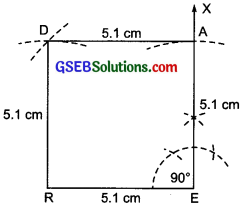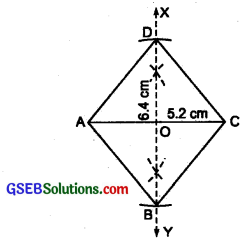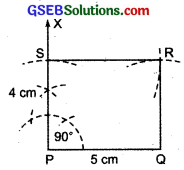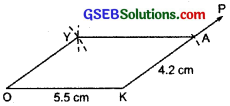# GSEB Solutions Class 8 Maths Chapter 4 Practical Geometry Ex 4.5

Gujarat Board GSEB Textbook Solutions Class 8 Maths Chapter 4 Practical Geometry Ex 4.5 Textbook Questions and Answers.

## Gujarat Board Textbook Solutions Class 8 Maths Chapter 4 Practical Geometry Ex 4.5

Question 1.
Draw the following.

1. The square READ with RE = 5.1 cm.
2. A rhombus whose diagonals are 5.2 cm and 6.4 cm long.
3. A rectangle with adjacent sides of lengths 5 cm and 4 cm.
4. A parallelogram OKAY where OK = 5.5 cm and KA = 4.2 cm.Solution:
1. Steps of construction:

I. Draw a line segment RE = 5.1 cm.
II. At E, draw $$\vec{EX}$$, such that ∠REX = 90°.
III. From $$\vec{EX}$$, cut-off $$\vec{EA}$$ = 5.1 cm.
IV. With centre at A, draw an arc above RE of radius = 5.1 cm.
V. With centre at R, and radius = 5.1 cm, draw another arc to intersect the previous arc at D.
VI. Join DA and DR. Thus, READ is the required square.2. Steps of construction:

I. Draw a line segment AC = 5.2 cm.
II. Draw $$\vec{XY}$$, the perpendicular bisector of AC.
III. From XY, cut-off OD = $$\frac{1}{2}$$(6.4 cm) = 3.2 cm.
IV. Similarly, cut-off OB = $$\frac{1}{2}$$ (6.4 cm) = 3.2 cm.
V. Join AD, DC, CB, and BA.
Thus, ABCD is the required rhombus.Note:
The diagonals of a rhombus bisect each other at right angles.

3. Steps of construction:

I. Draw a line segment PQ = 5 cm.
II. At P, draw $$\vec{PX}$$, such that ∠QPX = 90°.III. From $$\vec{PX}$$, cut – off PS = 4 cm.
IV. With centre at S and radius = 5 cm, mark an arc towards Q.
V. With centre Q and radius 4 cm, mark an arc to intersect the previous arc at R.
VI. Join RQ and RS
Thus, PQRS is the required rectangle.4. Steps of construction:

I. Draw a line segment OK = 5.5 cm.
II. At K, draw a ray $$\vec{KP}$$.
III. From $$\vec{KP}$$, cut – off KA = 4.2 cm.
IV. With centre at A and radius = 5.5 cm, draw an arc above OK.
V. With centre O and radius = 4.2 cm, draw another arc to intersect the previous arc at Y.VI. Join YO and YA.
Thus, OKAY is the required parallelogram.